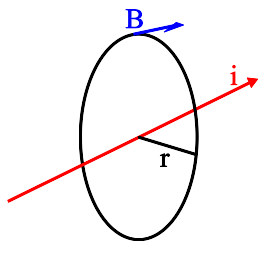# Field Due to an Infinite Straight Current Filament¶

This simple formula can be derived directly from Maxwell's fourth equation, or Ampere's Law.$B = \frac {\mu_o i}{2\pi r}$

B is the magnetic field, in teslas. The direction of the field is tangent to a circle on a radius r (in meters) from the wire.

$\mathbf \mu_o$ is the permeability constant (1.26x10-6 Hm-1)

i is the current in the wire, in amperes.

Magnet Formulas, © 2018 by Eric Dennison. Source code and License on Github